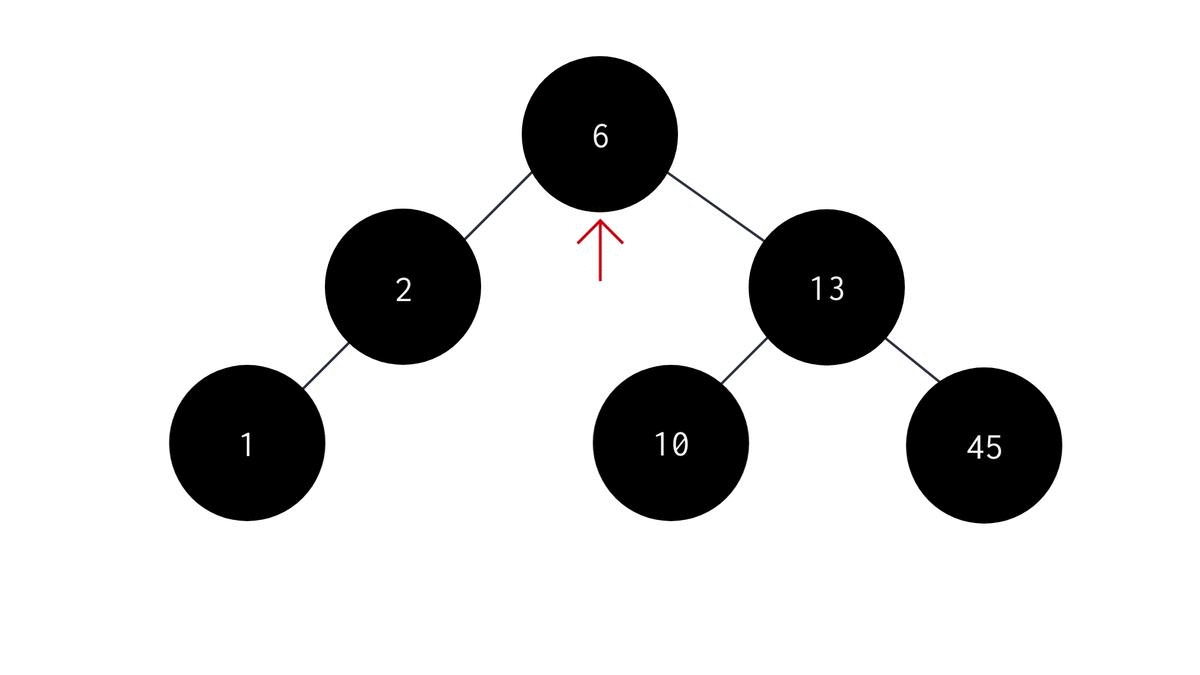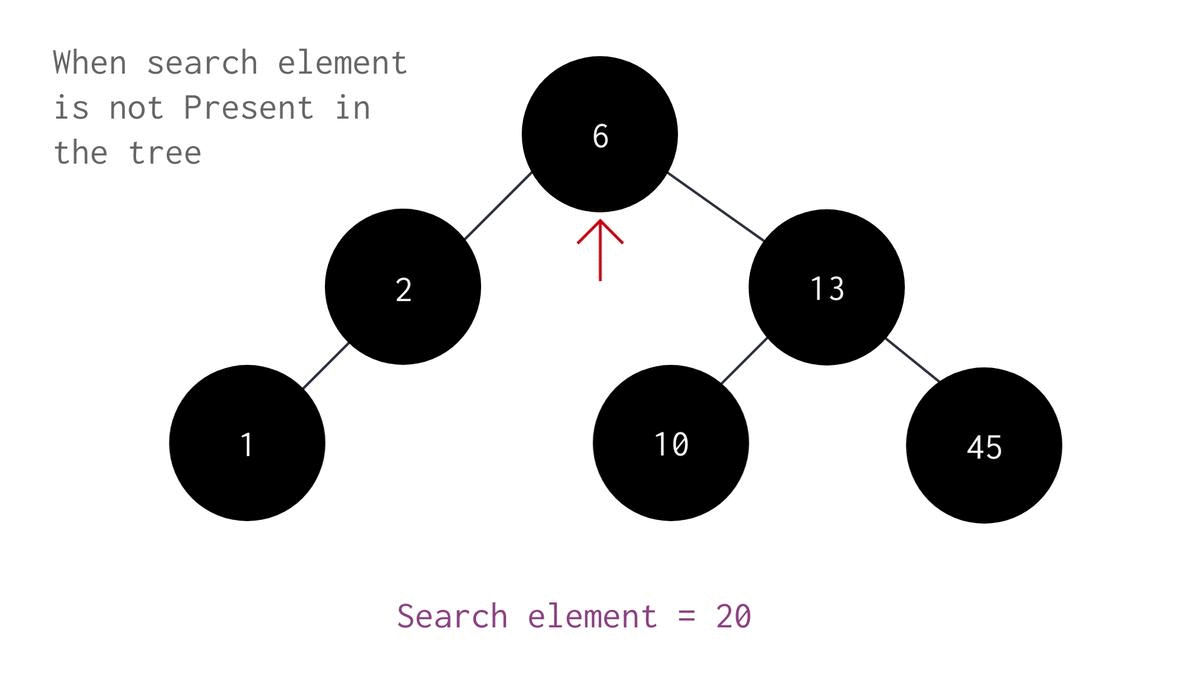# Searching in Binary Search TreeCreated July 20, 2021

In this post, I'm gonna be talking about how we can search for an element in a Binary Search Tree.

It is very easy to search for an element in a Binary Search Tree, easier than even in an array. All you have to do is compare the element's value with the value you're searching for at each level until you reach the targeted element.

## Algorithm

The algorithm for it looks something like this -

1. Start from the root element and check if it is empty or not. If it is empty then print "Empty tree". Else, do the following:
2. Compare the value that's being searched with the root node's value.
3. If its value is greater than the root node's value:
4. Repeat the steps from 1 to 2, replacing root with its right child(recursion by replacing root with its right child node).
5. If its value is less than the root node's value:
6. Repeat the steps from 1 to 2, replacing root with its left child(recursion by replacing root with its left child node).
7. Do the above until the targeted node is reached. If, you reach a leaf node, it means - the targeted value was not found in the tree.

## Code

The code for the above process is as follows:

``````void search(NODE *root, int val){
if(root == NULL){
printf("Empty Tree!\n");
}
else if(root->data != val && (root->left == NULL && root->right == NULL)){
}
else{
if(root->data == val){
printf("Value Found!\n");
}
else if(root->data > val){
//search in the left subtree
search(root->left, val);
}
else{
//search in the right subtree
search(root->right, val);
}
}
}
``````

Code for the whole process -

``````#include <stdio.h>
#include <stdlib.h>

typedef struct node{
int data;
struct node *left;
struct node *right;

}NODE;
NODE *createnode(int val){
NODE *newnode = (NODE *)malloc(sizeof(NODE));
newnode->data = val;
newnode->left = NULL;
newnode->right = NULL;
return newnode;
}
NODE *insertInTree(int val, NODE *root){
NODE *newnode = createnode(val);
if(root  == NULL){
root = newnode;
}else{
if(newnode->data <= root->data){
if(root->left == NULL){
root->left = newnode;
}else{
insertInTree(val, root->left);
}
}
else if(newnode->data > root->data){
if(root->right == NULL){
root->right = newnode;
}
else{
insertInTree(val, root->right);
}
}
}
return root;
}

void search(NODE *root, int val){
if(root == NULL){
printf("Empty Tree!\n");
}
else if(root->data != val && (root->left == NULL && root->right == NULL)){
}
else{
if(root->data == val){
printf("Value Found!\n");
}
else if(root->data > val){
//search in the left subtree
search(root->left, val);
}
else{
//search in the right subtree
search(root->right, val);
}
}
}
void inorder(NODE *root){
if(root == NULL){
return;
}else{
inorder(root->left);
printf("%d ", root->data);
inorder(root->right);
}
}

int main(){
NODE *root = NULL;
int ch=1;
printf("\tInsertion Operation\n\n");
do{
switch(ch){
case 1:
{
//insert
printf("Enter value for insertion: ");
int val;
scanf(" %d", &val);
root = insertInTree(val, root);
break;
}
case 0:
{
//Exit
printf("Exiting...\n");
break;
}
default:
{
printf("Enter valid choice!\n");
break;
}
}

printf("Enter:\n\t1->Continue\n\t0->Exit Insertion\n");
printf("Enter choice: ");
scanf(" %d", &ch);
}while(ch != 0);

printf("Inorder representation: ");
inorder(root);
printf("\n");

printf("\n\n\tSearch Operation\n\n");
ch=1;
do{
switch(ch){
case 1:
{
//insert
printf("Enter value for searching: ");
int val;
scanf(" %d", &val);
search(root, val);
break;
}
case 0:
{
//Exit
printf("Exiting...\n");
break;
}
default:
{
printf("Enter valid choice!\n");
break;
}
}

printf("Enter:\n\t1->Continue\n\t0->Exit Searching\n");
printf("Enter choice: ");
scanf(" %d", &ch);
}while(ch != 0);

}
``````

Here, we've first used the "insertInTree" function to build the binary tree, and then we've used the "search" function to search the user entered value. Here's exactly what the above code is doing -